# Weekly Export Inspections of Soybeans during the 2018 Trade War with China

Photo by Ronan on Unsplash

All eyes are on export sales and shipments of soybeans in the midst of the 2018 trade war.

I always have my commodity price analysis students produce those ‘export pace of use’ charts as a lab assignment because it is data that is very actively followed in the commodity marketing business and because I get to teach them how to use pivot tables in Excel. I’ve had a hankering for awhile to produce the same graphs with tidyverse functions in R, and this year I am especially interested in following the progress of export sales and shipments to see how the trade war is playing out. But I’m not excited about babysitting the excel spreadsheets and manually copy and pasting the new data, so now is a good time to go ahead and do this exercise in R!

First, I’ll just present graphs, and below I will provide the code to reproduce the charts on your own if you want. I’ll be updating them frequently for the next few weeks for my own interest.

I’m going to use the export inspection data from the USDA Federal Grain Inspection Services (FGIS) rather than the more commonly followed Foreign Aricultural Service (FAS) export sales data. Mostly this is because its what I use in my class, the FGIS provides every export inspection as a datapoint in a .csv file, and gives us the opportunity to do a little more intense data work.

Since ‘sales’ and ‘export inspections’ at the port do not happen at the same time, the graphs in this post look different than what you typcially see tweeted after the FAS report comes out on Thursdays. The FAS report gives us information faster because we know about sales within one week (or one day for the big sales). However, sometimes these sales are cancelled before they get shipped, so the FGIS data is exports that we know left the ports (this is my understanding anyway).

First the weekly export inspections for soybeans in 2018 compared to 2013-2017.

The last couple of weeks’ export inspections have been the worst since 2013.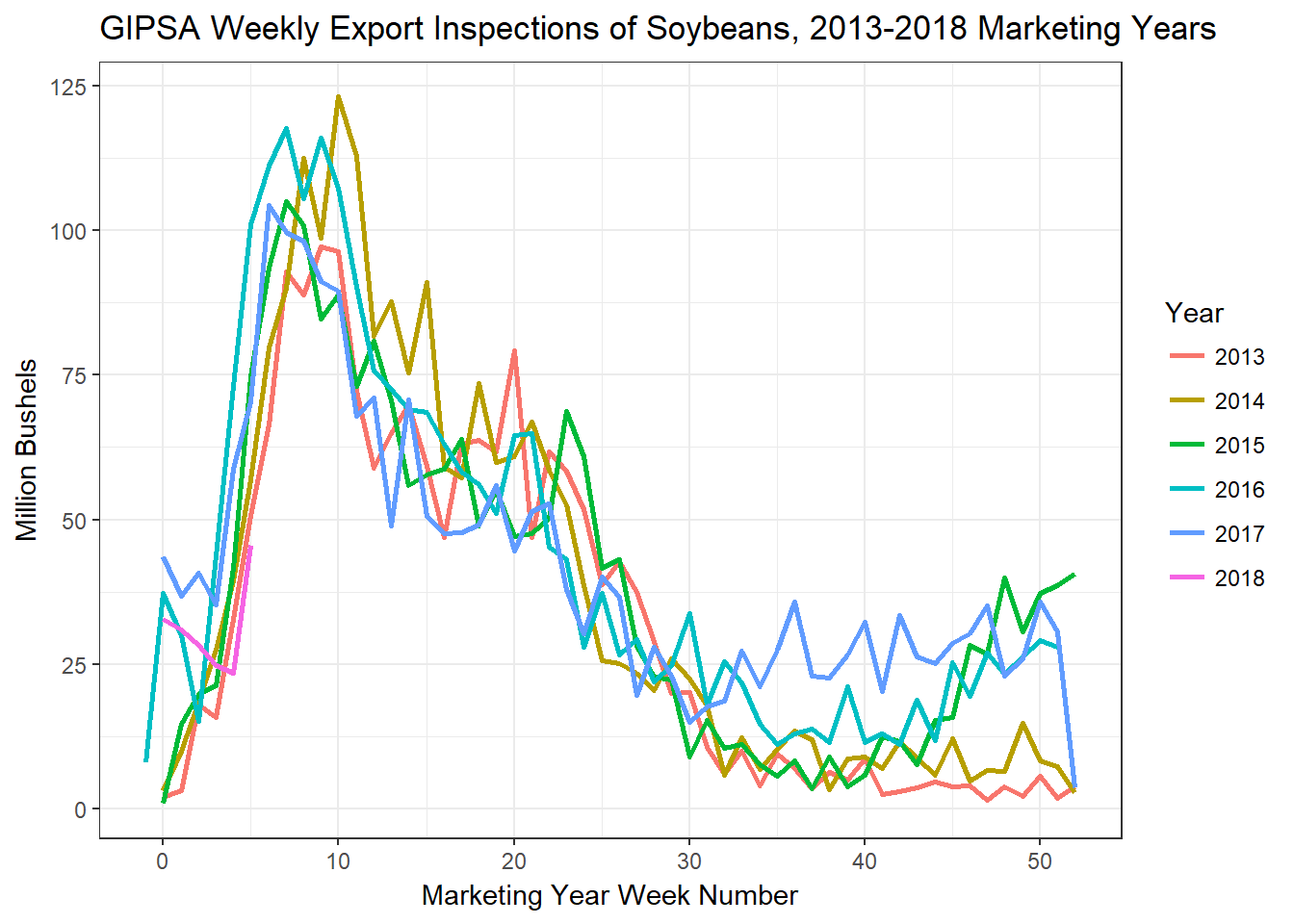Looking at the cumulative inspections for the 2018 marketing year, export shipments of soybeans are off to the worst start since 2015.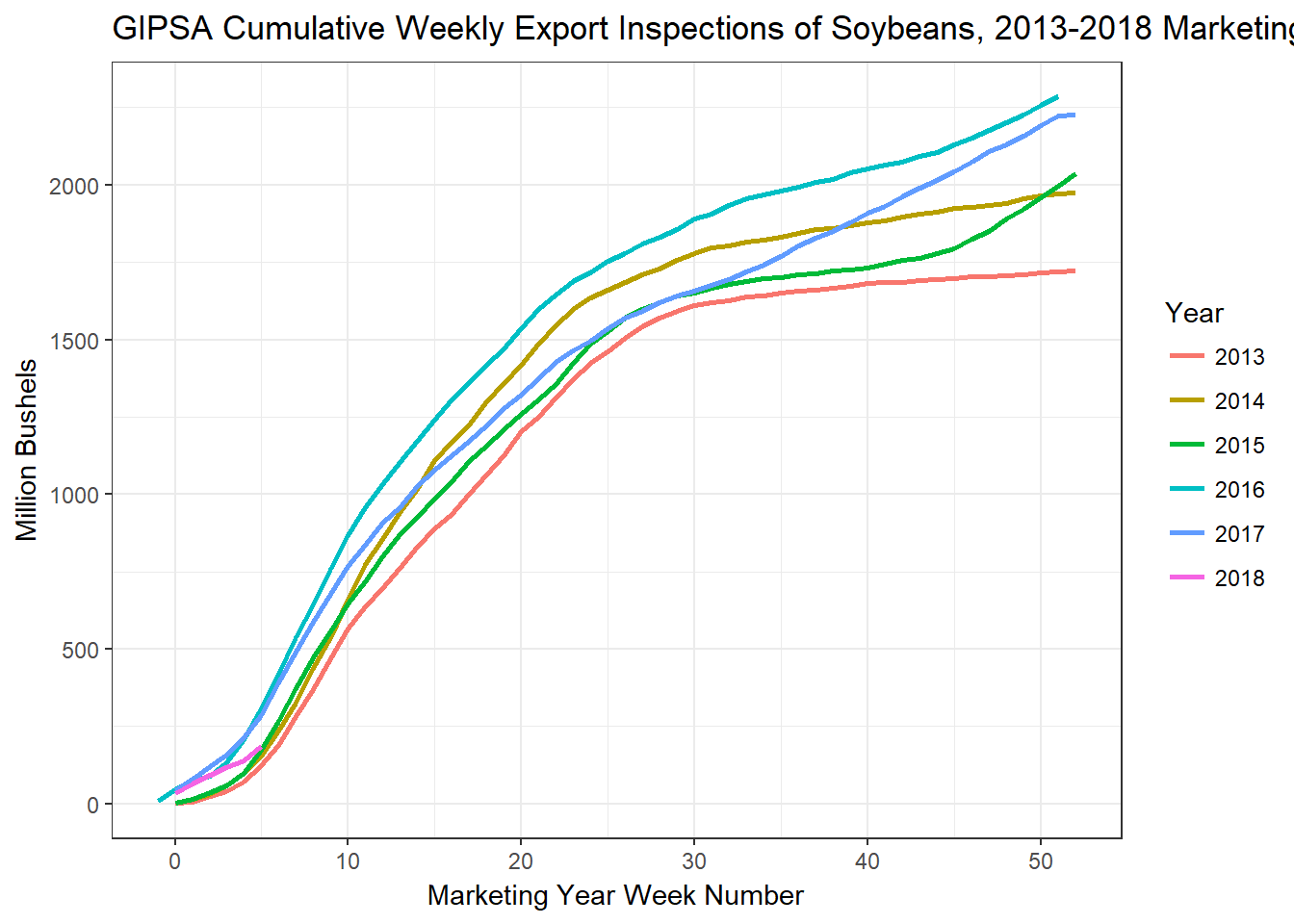As I said, this data is behind what we know from the FAS export sales report and this weeks sales were ‘disappointing’. INTL FCStone had this assessment:

So we can expect the export inspections to keep coming in weak unless something changes fast.

Of course, it is no mystery that the trade war with China is the reason for this. If we plot the week 1-6 export inspections for China since 2013, 2018 is the tiny pink bars in week’s 1 and 6. There were no export inspections to China in weeks 2-5.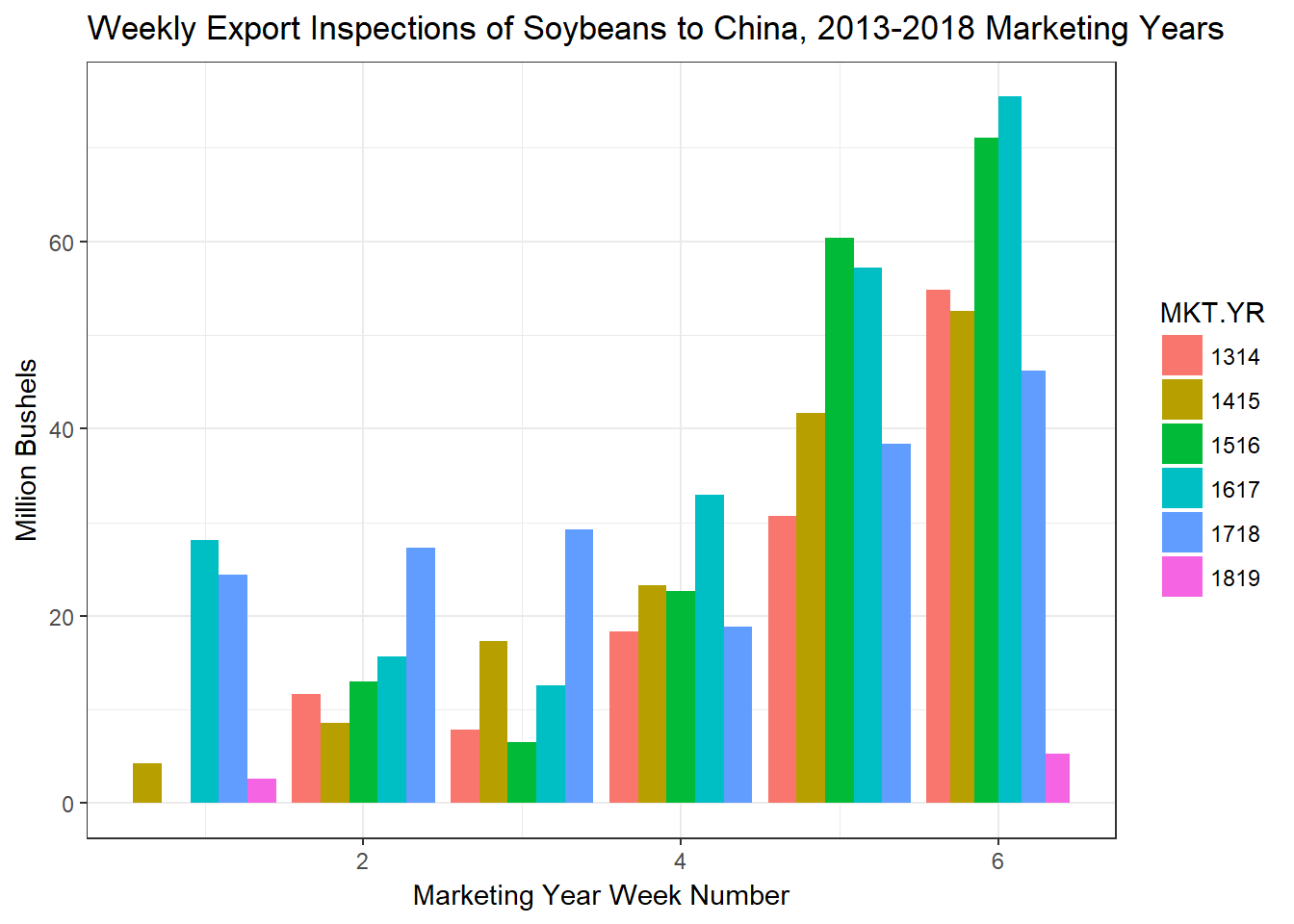Most think that China will eventually step in and buy a significant amount of soybeans just based on their raw needs and the fact that Brazil should be just about out of their last harvest. Todd Hubbs gives and excellent analysis of this in a recent FarmDoc Daily article. As Todd identifies, the big question is what price will it take for Chinese buyers to come back to the U.S.? We are $2 off the spring price and the premium at the Brazilian ports has never been higher, yet shipments to China still haven’t materialized. For context here is the cumulative weekly export inspections for corn.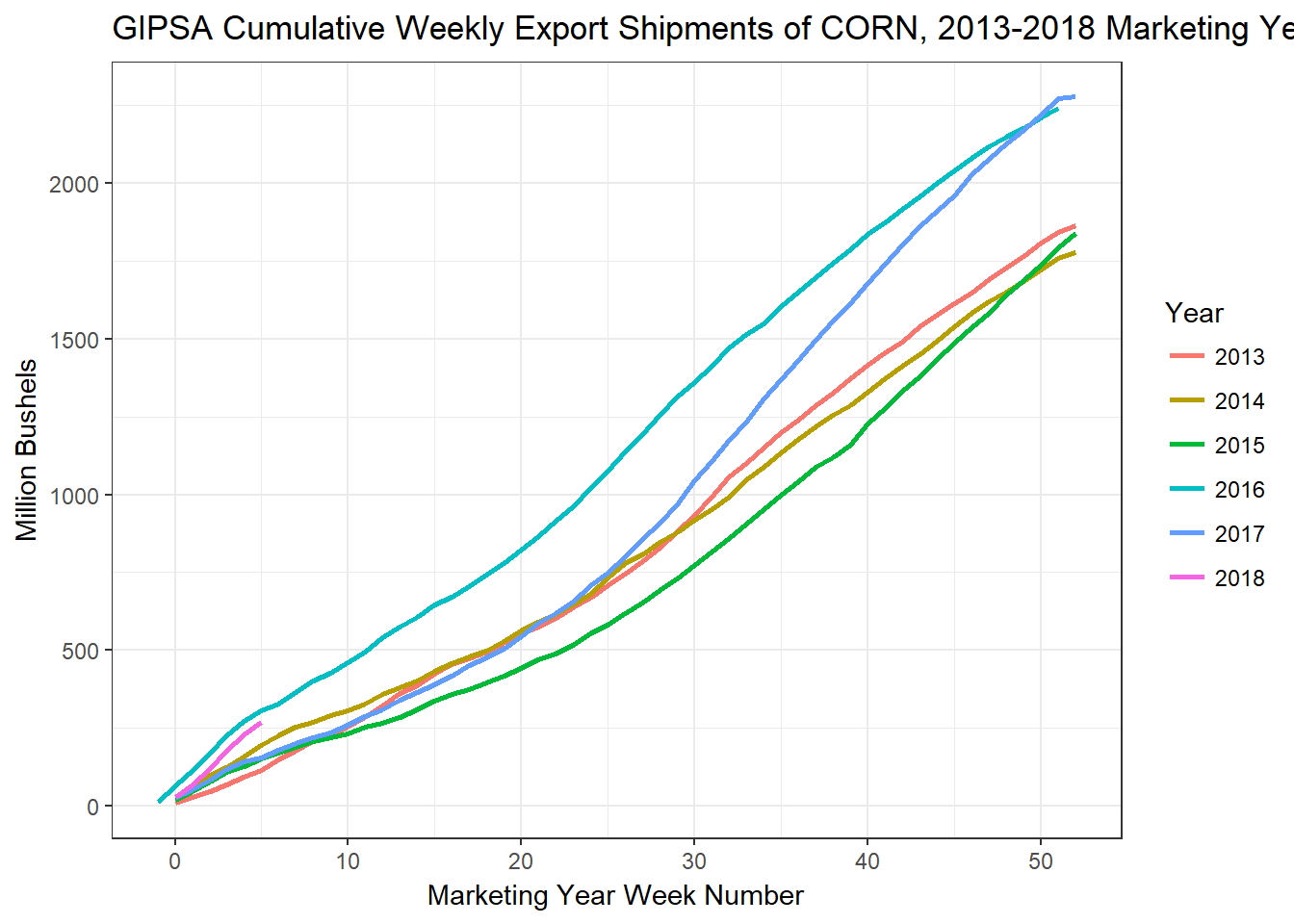And for wheat (all varieties).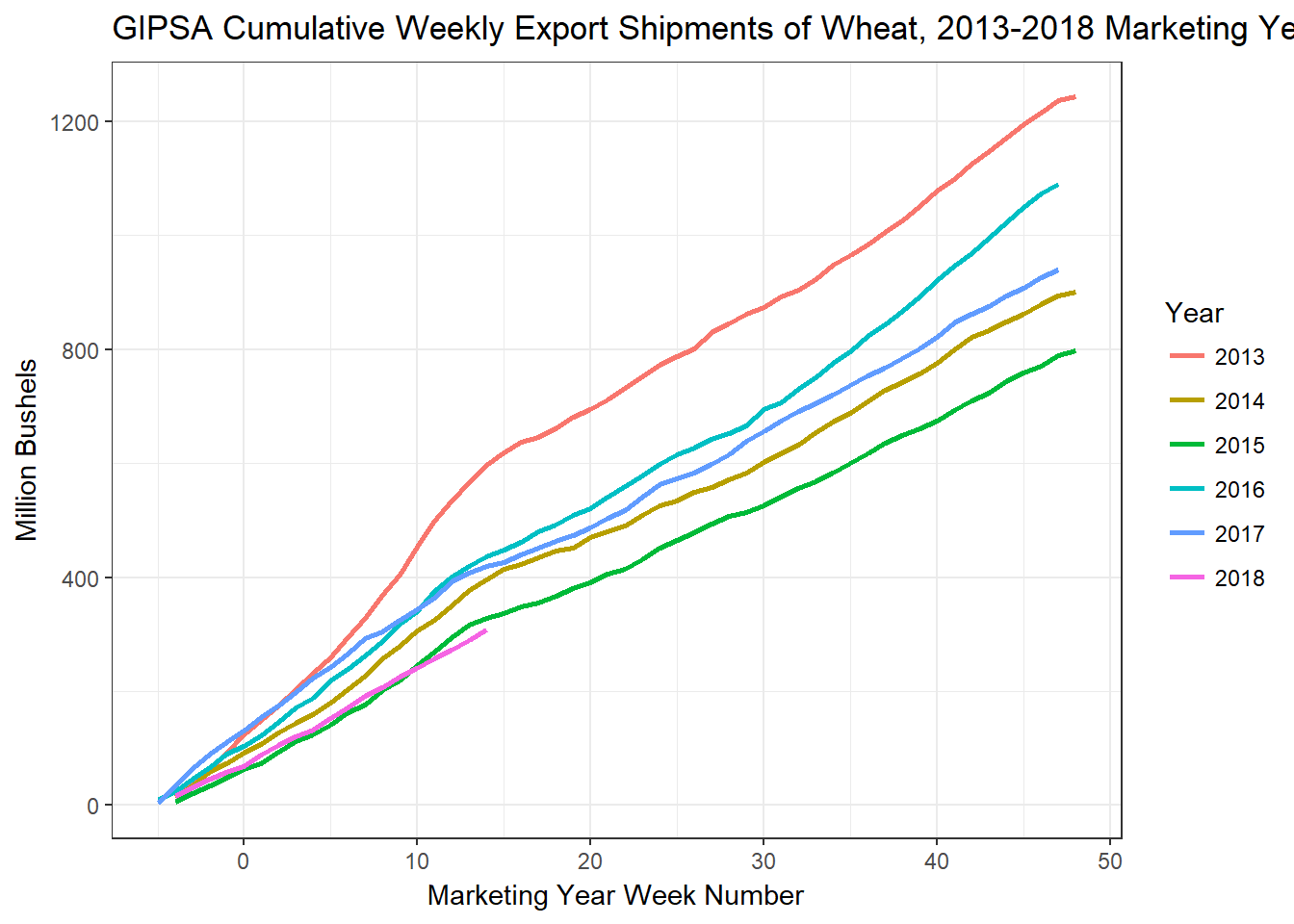The code to reproduce (and therefore update!) all of the charts yourself is below. library(tidyverse) # Get the data from GIPSA DATA_2018 <- read.csv(file = "https://fgisonline.ams.usda.gov/ExportGrainReport/CY2018.csv") DATA_2017 <- read.csv(file = "https://fgisonline.ams.usda.gov/ExportGrainReport/CY2017.csv") DATA_2016 <- read.csv(file = "https://fgisonline.ams.usda.gov/ExportGrainReport/CY2016.csv") DATA_2015 <- read.csv(file = "https://fgisonline.ams.usda.gov/ExportGrainReport/CY2015.csv") DATA_2014 <- read.csv(file = "https://fgisonline.ams.usda.gov/ExportGrainReport/CY2014.csv") DATA_2013 <- read.csv(file = "https://fgisonline.ams.usda.gov/ExportGrainReport/CY2013.csv") DATA <- rbind(DATA_2013, DATA_2014, DATA_2015, DATA_2016, DATA_2017, DATA_2018) DATA <- DATA %>% select(Thursday, Grain, Pounds, Destination, MKT.YR) %>% separate(col = 'Thursday', sep = c(4, 6), into = c('Year', 'Month', 'Day'), remove = TRUE, convert = FALSE) %>% unite(col = 'Date', Year, Month, Day, sep = '-', remove = FALSE) DATA$Date <- as.Date(DATA$Date) DATA$Pounds <- DATA$Pounds/56/1000000 # Converts lbs to million bu ###################################### # For soybeans # If a tidyverse person reads this: I have to separate out marketing years because if I keep the # data in one big dataframe and some over weeks since 09-01 I will mix crop years because right # when harvest begins both new crop and old crop are being exported. In the raw .csv you will see # for the same week different observations can be from different marketing years and summing them # together would be a mistake. If there is a more elegant solution, I am all ears. # 2013 soy_13 <- filter(DATA, Grain == "SOYBEANS" & MKT.YR == '1314') %>% mutate(MkYrWk = floor(difftime(Date, '2013-09-01', units = "weeks"))) %>% # Calculate the week of the marketing year group_by(MkYrWk) %>% summarize(WkTotal = sum(Pounds)) %>% # Sum total pounds inspected each week of the mkting year mutate(WkCumSum = cumsum(WkTotal)) # Calculate a cumulative sum of pounds inspected by week. soy_13$Year <- '2013'                  # Attach a year identifier for when we paste the years back together

# 2014
soy_14 <- filter(DATA, Grain == "SOYBEANS" & MKT.YR == '1415') %>%
mutate(MkYrWk = floor(difftime(Date, '2014-09-01', units = "weeks"))) %>%
group_by(MkYrWk) %>%
summarize(WkTotal = sum(Pounds)) %>%
mutate(WkCumSum = cumsum(WkTotal))

soy_14$Year <- '2014' # 2015 soy_15 <- filter(DATA, Grain == "SOYBEANS" & MKT.YR == '1516') %>% mutate(MkYrWk = floor(difftime(Date, '2015-09-01', units = "weeks"))) %>% group_by(MkYrWk) %>% summarize(WkTotal = sum(Pounds)) %>% mutate(WkCumSum = cumsum(WkTotal)) soy_15$Year <- '2015'

# 2016
soy_16 <- filter(DATA, Grain == "SOYBEANS" & MKT.YR == '1617') %>%
mutate(MkYrWk = floor(difftime(Date, '2016-09-01', units = "weeks"))) %>%
group_by(MkYrWk) %>%
summarize(WkTotal = sum(Pounds)) %>%
mutate(WkCumSum = cumsum(WkTotal))

soy_16$Year <- '2016' # 2017 soy_17 <- filter(DATA, Grain == "SOYBEANS" & MKT.YR == '1718') %>% mutate(MkYrWk = floor(difftime(Date, '2017-09-01', units = "weeks"))) %>% group_by(MkYrWk) %>% summarize(WkTotal = sum(Pounds)) %>% mutate(WkCumSum = cumsum(WkTotal)) soy_17$Year <- '2017'

# 2018
soy_18 <- filter(DATA, Grain == "SOYBEANS" & MKT.YR == '1819') %>%
mutate(MkYrWk = floor(difftime(Date, '2018-09-01', units = "weeks"))) %>%
group_by(MkYrWk) %>%
summarize(WkTotal = sum(Pounds)) %>%
mutate(WkCumSum = cumsum(WkTotal))

soy_18$Year <- '2018' soy <- rbind(soy_13, soy_14, soy_15, soy_16, soy_17, soy_18) soy$MkYrWk <- as.numeric(soy$MkYrWk) soy$Year  <- as.factor(soy$Year) # Soy plots ggplot(soy, aes(x = MkYrWk, y = WkTotal, color = Year)) + geom_line(size = 1) + theme_bw() + labs(title = "GIPSA Weekly Export Inspections of Soybeans, 2013-2018 Marketing Years", x = 'Marketing Year Week Number', y = 'Million Bushels') ggplot(soy, aes(x = MkYrWk, y = WkCumSum, color = Year)) + geom_line(size = 1) + theme_bw() + labs(title = "GIPSA Cumulative Weekly Export Inspections of Soybeans, 2013-2018 Marketing Years", x = 'Marketing Year Week Number', y = 'Million Bushels') # Prep data to look at China's inspections only soy_China <- filter(DATA, Grain == "SOYBEANS" & Destination == 'CHINA MAIN') %>% mutate(MkYrWk = as.numeric(floor(difftime(Date, '2013-09-01', units = "weeks")))%% 52) %>% unite(col = 'MYMkYrWk', MKT.YR, MkYrWk, sep = '', remove = TRUE) %>% group_by(MYMkYrWk) %>% summarize(WkTotal = sum(Pounds)) %>% mutate(WkCumSum = cumsum(WkTotal)) %>% separate(col = 'MYMkYrWk', sep = c(4), into = c('MKT.YR', 'MkYrWk'), remove = TRUE, convert = FALSE) soy_China$MkYrWk <- as.numeric(soy_China$MkYrWk) soy_China <- filter(soy_China, MkYrWk %in% (1:6)) ggplot(soy_China, aes(x = MkYrWk, y = WkTotal, fill = MKT.YR)) + geom_bar(stat = "identity", position = "dodge") + theme_bw() + labs(title = "Weekly Export Inspections of Soybeans to China, 2013-2018 Marketing Years", x = 'Marketing Year Week Number', y = 'Million Bushels') #################################################### # For Corn # 2013 corn_13 <- filter(DATA, Grain == "CORN" & MKT.YR == '1314') %>% mutate(MkYrWk = floor(difftime(Date, '2013-09-01', units = "weeks"))) %>% group_by(MkYrWk) %>% summarize(WkTotal = sum(Pounds)) %>% mutate(WkCumSum = cumsum(WkTotal)) corn_13$Year <- '2013'

# 2014
corn_14 <- filter(DATA, Grain == "CORN" & MKT.YR == '1415') %>%
mutate(MkYrWk = floor(difftime(Date, '2014-09-01', units = "weeks"))) %>%
group_by(MkYrWk) %>%
summarize(WkTotal = sum(Pounds)) %>%
mutate(WkCumSum = cumsum(WkTotal))

corn_14$Year <- '2014' # 2015 corn_15 <- filter(DATA, Grain == "CORN" & MKT.YR == '1516') %>% mutate(MkYrWk = floor(difftime(Date, '2015-09-01', units = "weeks"))) %>% group_by(MkYrWk) %>% summarize(WkTotal = sum(Pounds)) %>% mutate(WkCumSum = cumsum(WkTotal)) corn_15$Year <- '2015'

# 2016
corn_16 <- filter(DATA, Grain == "CORN" & MKT.YR == '1617') %>%
mutate(MkYrWk = floor(difftime(Date, '2016-09-01', units = "weeks"))) %>%
group_by(MkYrWk) %>%
summarize(WkTotal = sum(Pounds)) %>%
mutate(WkCumSum = cumsum(WkTotal))

corn_16$Year <- '2016' # 2017 corn_17 <- filter(DATA, Grain == "CORN" & MKT.YR == '1718') %>% mutate(MkYrWk = floor(difftime(Date, '2017-09-01', units = "weeks"))) %>% group_by(MkYrWk) %>% summarize(WkTotal = sum(Pounds)) %>% mutate(WkCumSum = cumsum(WkTotal)) corn_17$Year <- '2017'

# 2018
corn_18 <- filter(DATA, Grain == "CORN" & MKT.YR == '1819') %>%
mutate(MkYrWk = floor(difftime(Date, '2018-09-01', units = "weeks"))) %>%
group_by(MkYrWk) %>%
summarize(WkTotal = sum(Pounds)) %>%
mutate(WkCumSum = cumsum(WkTotal))

corn_18$Year <- '2018' corn <- rbind(corn_13, corn_14, corn_15, corn_16, corn_17, corn_18) corn$MkYrWk <- as.numeric(corn$MkYrWk) corn$Year  <- as.factor(corn$Year) ggplot(corn, aes(x = MkYrWk, y = WkCumSum, color = Year)) + geom_line(size = 1) + theme_bw() + labs(title = "GIPSA Cumulative Weekly Export Shipments of CORN, 2013-2018 Marketing Years", x = 'Marketing Year Week Number', y = 'Million Bushels') # For wheat # 2013 wheat_13 <- filter(DATA, Grain == "WHEAT" & MKT.YR == '1314') %>% mutate(MkYrWk = floor(difftime(Date, '2013-07-01', units = "weeks"))) %>% group_by(MkYrWk) %>% summarize(WkTotal = sum(Pounds)) %>% mutate(WkCumSum = cumsum(WkTotal)) wheat_13$Year <- '2013'

# 2014
wheat_14 <- filter(DATA, Grain == "WHEAT" & MKT.YR == '1415') %>%
mutate(MkYrWk = floor(difftime(Date, '2014-07-01', units = "weeks"))) %>%
group_by(MkYrWk) %>%
summarize(WkTotal = sum(Pounds)) %>%
mutate(WkCumSum = cumsum(WkTotal))

wheat_14$Year <- '2014' # 2015 wheat_15 <- filter(DATA, Grain == "WHEAT" & MKT.YR == '1516') %>% mutate(MkYrWk = floor(difftime(Date, '2015-07-01', units = "weeks"))) %>% group_by(MkYrWk) %>% summarize(WkTotal = sum(Pounds)) %>% mutate(WkCumSum = cumsum(WkTotal)) wheat_15$Year <- '2015'

# 2016
wheat_16 <- filter(DATA, Grain == "WHEAT" & MKT.YR == '1617') %>%
mutate(MkYrWk = floor(difftime(Date, '2016-07-01', units = "weeks"))) %>%
group_by(MkYrWk) %>%
summarize(WkTotal = sum(Pounds)) %>%
mutate(WkCumSum = cumsum(WkTotal))

wheat_16$Year <- '2016' # 2017 wheat_17 <- filter(DATA, Grain == "WHEAT" & MKT.YR == '1718') %>% mutate(MkYrWk = floor(difftime(Date, '2017-07-01', units = "weeks"))) %>% group_by(MkYrWk) %>% summarize(WkTotal = sum(Pounds)) %>% mutate(WkCumSum = cumsum(WkTotal)) wheat_17$Year <- '2017'

# 2018
wheat_18 <- filter(DATA, Grain == "WHEAT" & MKT.YR == '1819') %>%
mutate(MkYrWk = floor(difftime(Date, '2018-07-01', units = "weeks"))) %>%
group_by(MkYrWk) %>%
summarize(WkTotal = sum(Pounds)) %>%
mutate(WkCumSum = cumsum(WkTotal))

wheat_18$Year <- '2018' wheat <- rbind(wheat_13, wheat_14, wheat_15, wheat_16, wheat_17, wheat_18) wheat$MkYrWk <- as.numeric(wheat$MkYrWk) wheat$Year  <- as.factor(wheat\$Year)

ggplot(wheat, aes(x = MkYrWk, y = WkCumSum, color = Year)) +
geom_line(size = 1) +
theme_bw() +
labs(title = "GIPSA Cumulative Weekly Export Shipments of Wheat, 2013-2018 Marketing Years", x = 'Marketing Year Week Number', y = 'Million Bushels')